# Catch up on the latest AI articles# When, Why, And Which Pretrained GANs Are Useful?

3 main points
✔️ Investigate the success of pre-trained GANs
✔️ Explain the role of Generator and Discriminator in pre-trained GANs
✔️ Propose policies for optimal source dataset selection

written by Timofey GrigoryevAndrey VoynovArtem Babenko
(Submitted on 17 Feb 2022 (v1), last revised 10 Mar 2022 (this version, v2))

Subjects:  Machine Learning (cs.LG); Computer Vision and Pattern Recognition (cs.CV)code：The images used in this article are from the paper, the introductory slides, or created based on them.

## first of all

Recent work has shown that fine-tuning a pre-trained GAN on a different dataset (especially in the case of small numbers of data) gives better results than training from scratch.

So when, why, and how do pre-trained GANs perform better?

In this article, we have analyzed various aspects of the above questions, such as how the use of pre-trained GANs affects the generated images, what role the initialization of Generator and Discriminator plays, and what pre-trained GAN to choose for the desired task We will introduce our research.

## Analysis of GAN fine-tuning

To begin, we analyze why GAN fine-tuning shows superior results compared to learning from scratch.

### Intuitive predictions of why GAN fine-tuning is successful

First, consider the case of fine-tuning with new data from the distribution $p_{target}$ for the pre-trained Generator and Discriminator$G, D$.

In the paper, we predict that the pre-learned $G, D$ play the following roles, respectively, and show by experiment that this prediction is likely to be correct.

• Generator initialization is responsible for modes coverage of target data.
• The initialization of the Discriminator is responsible for the initial gradient field.

If based on this expectation, the reason for success by using pre-trained $G, D$ can be expressed as follows.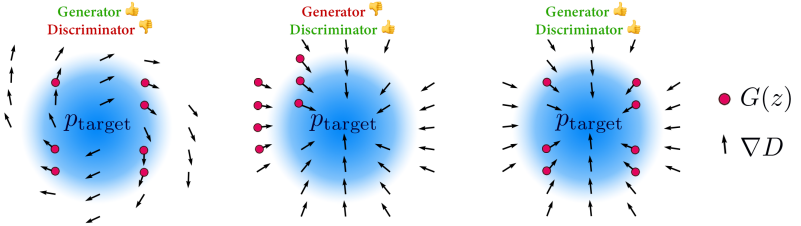In the left part of the figure, you can see an image of what happens when you use the appropriate Generator and the inappropriate Discriminator.

In this case, the Generator is generating diverse initial samples, but the learning is not performing well because the gradient given by the Discriminator is not favorable. In the middle of the figure, an image of the case with an inappropriate Generator and an appropriate Discriminator is shown.

In this case, the gradient given by the Discriminator is good, but the distribution of the initial samples generated by the Generator is not adequate, which would bias the final generated mode range. And as shown on the right side of the figure, if both the Generator and the Discriminator are appropriate, then we can assume that we can properly transition to the new task.

In the following sections, we will perform experiments on synthetic data to confirm the validity of this prediction.

### Experiments with synthetic data

In our experiments, we consider the synthetic data presented in the following figure.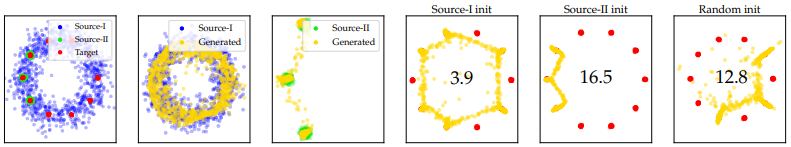The first from the left in the figure shows the two source data (Source-I and Source-II) and the target data (Target).

The second and third figures show examples of pre-trained Generator generation on Source-I and Source-II, respectively.

The results for these two pre-trained Generators and for training the Generator from scratch on the target data are shown in Figures 4, 5, and 6, respectively (the numbers in the figures are the target distribution and the generated data Wasserstein-1).

As shown in the figure, we have shown that using an appropriate pre-trained Generator that generates a variety of data gives better results compared to the from-scratch case. (Conversely, we also found that using a generator with a biased set of generated examples leads to worse results than the from the scratch case.)

We further investigate how the choice of pre-trained Generator and Discriminator affects the results after fine-tuning.

Here, we measure the quality of the pre-trained Generator by Recall and the quality of the pre-trained Discriminator by the similarity between the Ground truth gradient and the Discriminator gradient.

For different pre-trained Generator and Discriminator pairs, we perform fine-tuning on the target data (same settings as in the previous experiment) and measure the Wasserstein-1 distance between the target data distribution and the generator distribution. With this procedure, we investigate the relationship between the quality of the pre-trained Generator and Discriminator and their final performance. The results are as follows.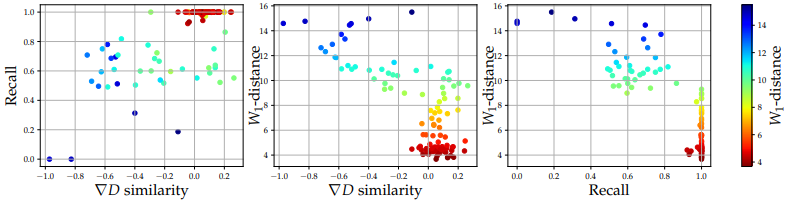On the left of the figure, the Recall and ∇D similarity of the selected Generator and Discriminator is plotted.

The center and right of the figure show the relationship between the quality of the Discriminator (∇D similarity) and Generator (Recall) and the quality of the generated result (W1-distance), respectively.

As can be seen from the results, the quality of the Generator and Discriminator is shown to be significantly negatively correlated with the quality of generation after fine-tuning (Pearson correlation coefficient is -0.84 for Recall and -0.73 for ∇D similarity).

The experiments show that the Recall and ∇D similarity of the pre-trained Generator and Discriminator is correlated with the quality of the GAN after fine-tuning. However, it should be noted that this does not prove causality.

## experimental setup

### Pre-study of StyleGAN2

In our experiments, we investigate the StyleGAN2 architecture.

Data Set

The datasets used for pre-training and targeting are as follows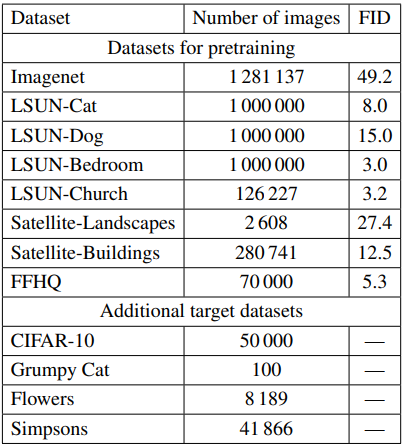In the table, we perform pre-training on the 8 datasets included in Datasets for pretraining and fine-tuning on the Additional target datasets. The image resolution is 256x256 and the model is the official PyTorch implementation of StyleGAN2-ADA. The FID in the table shows the FID score of the pre-trained model on each dataset, the smaller the better.

Pre-study settings

For the pre-trained models, we first create a model trained on 50M images in ImageNet, which is then trained on 25M images for each remaining dataset to create 7 checkpoints.

Training settings on the target dataset

For training on the target datasets, we use the default transfer training settings of the StyleGAN2-ADA implementation, with 25M images for each dataset.

Evaluation Indicators

The metrics used to evaluate the performance of the model are as follows

• FID
• Precision
• recall
• Convergence rate: An index that quantifies how quickly the learning process reaches a plateau (stable state).

## experimental results

The results of the experiment are as follows: F, P, R, and C denote FID, Precision, Recall, and Convergence rate respectively.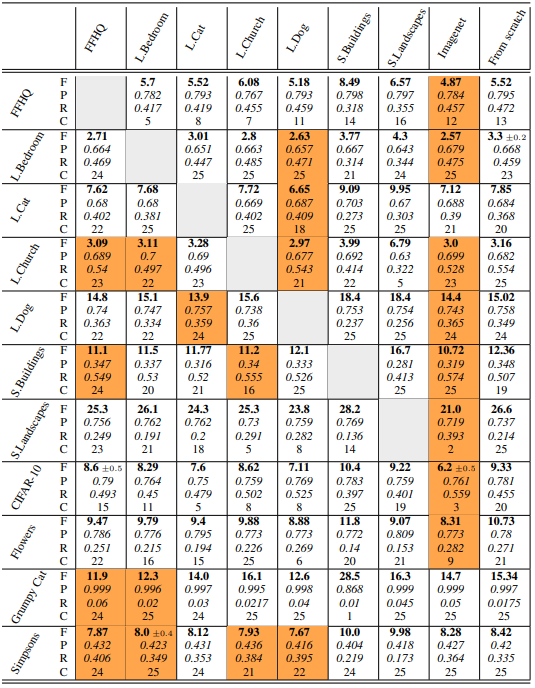From the table, we can see that

• For FID, pre-trained checkpoints on diverse source data (ImageNet, LSUN Dog) outperformed training from scratch (From scratch) on all datasets.
• Pre-learning significantly speeds up optimization compared to learning from scratch.
• The choice of source checkpoints has a significant effect on the Recall value of the fine-tuned model. For example, if the target is the Flowers dataset, the variation is over 10%.

The standard deviations of Recall and Precision for each target are as follows, and it is clear that the variation of Recall is still large.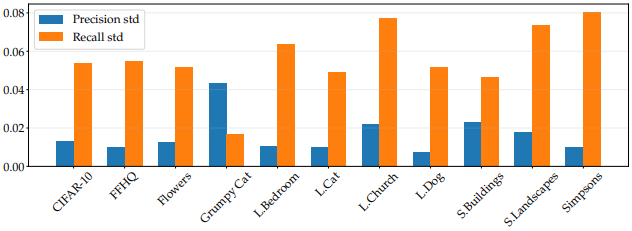In general, we found that despite the low FID score of the model (49.2), the model pre-trained with ImageNet is a good checkpoint for fine-tuning.

This result is contrary to previous studies, but this is probably due to the different models used (WGAN-GP).

Pre-Learning to Improve Mode Coverage of Real Data

The paper predicts that the initialization of the Generator is related to the mode coverage of the target data. Here, additional experiments investigate how much the choice of the pre-training model affects the mode of the generated images.

Specifically, for the generated images of the fine-tuned model on the Flowers dataset, which consists of 102 classes, we examine how many images within each class are present by the classifier. The results are as follows.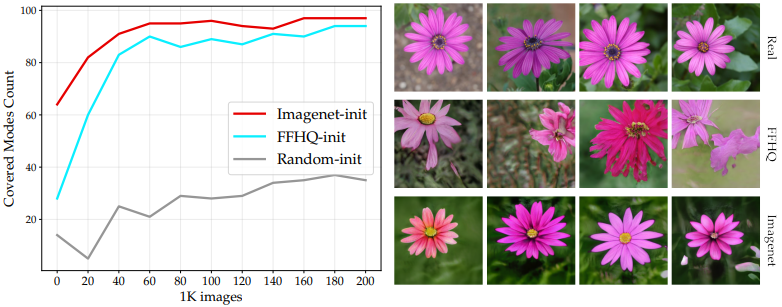In this figure, for each checkpoint (ImageNet, FFHQ, and Random), 10,000 images are generated and the number of classes containing 10 or more samples is plotted (the horizontal axis is the total number of trained images in the model).

As the figure shows, models pre-trained on ImageNet with diverse source data cover more diverse modes.

How to select appropriate pre-studied checkpoints

Finally, we introduce a simple policy on what pre-trained checkpoints should be selected for a particular target dataset.

Specifically, we consider selecting the best model by measuring the similarity of the distributions of the source and target datasets. Here, we measure FID, KID, Precision, and Recall for two cases: (1) simply using the images in the source and target datasets, and (2) using the generated examples of pre-trained models and the images in the target dataset.

The results of determining whether the best source dataset can be identified by the numerical value of each indicator are as follows.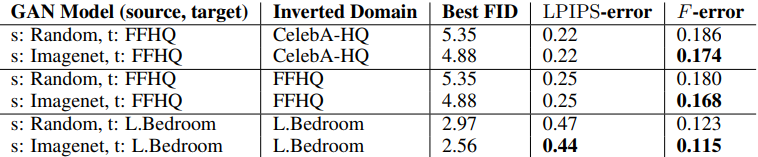The table shows the number of datasets that failed to predict the optimal checkpoint, with smaller numbers indicating a better indicator.

In general, we found that by using metrics other than Precision (especially Recall), we can roughly identify the optimal source dataset for both settings (1) and (2).

## summary

In this article, we presented a paper that investigated the success of pre-trained GAN models.

The paper presented various findings, including that the use of appropriate pre-trained GANs can improve the coverage of the modes of the generated images and that both pre-trained Generators and Discriminators should be used for optimal performance. We also found that the Recall value between source and target datasets can guide the selection of an appropriate source dataset.

If you have any suggestions for improvement of the content of the article,